# What is the Equation of a Circle?: Equation of a Circle Examples

• Author

Joao Amadeu has more than 10 years of experience in teaching physics and mathematics at different educational levels. Joao earned two degrees at Londrina State University: B.S. in Physics and M.S. in Science and Mathematics Education. He is currently working on his PhD in Science Education at Western Michigan University.

• Instructor
Lance Cain
This lesson focuses on defining a circle, presenting its equation in two different forms (standard and general), and giving examples of how to solve problems. Updated: 12/07/2021

Show

## What is the Equation of a Circle?

To explore the different forms that an equation of a circle can appear as it is important to understand what a circle and its parts are in mathematics. Basically, a circle is a curve that closes on itself and has vertical and horizontal symmetry.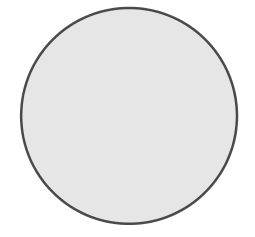A circle can be broken down into two parts: the circumference and center. The circumference is a sequence of points that are equidistant to the center, while the center is a point that marks the central location of the figure. The segment that connects any point of the circumference to the center is called the radius. Paraphrasing one of the postulates developed by Euclid, a circle can be drawn with line segments that have the length equal to the radius of the circle, and that have the center of the circle as an endpoint.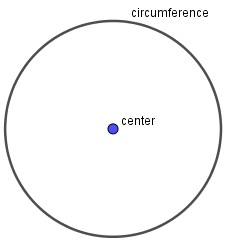A simple explanation of the equation of a circle is that it is mathematical equality that generates specific circles. These equations allow one to draw these figures on a coordinate system (x and y-axis).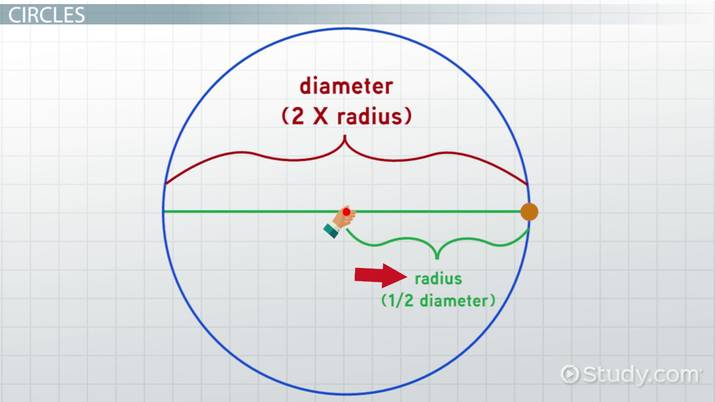An error occurred trying to load this video.

Try refreshing the page, or contact customer support.

Coming up next: Radian Measure: Definition & Formula

### You're on a roll. Keep up the good work!

Replay
Your next lesson will play in 10 seconds
• 0:04 Circles
• 1:45 Standard Form Vs General Form
• 2:37 Some Examples
• 8:08 Lesson Summary
Save Save

Want to watch this again later?

Timeline
Autoplay
Autoplay
Speed Speed

## How to Find the Equation of a Circle

To understand how to find the equation of a circle, one needs to be familiar with the Pythagorean Theorem (hypotenuse formula). This theorem is applied to right triangles that, in the case of a circle, have their hypotenuse formed by a segment drawn from the center of a circle to a point in the circumference (radius).

The following sections will go in-depth in using the hypothenuse formula to explore circle equations.

### Hypotenuse Formula

The hypotenuse formula is based on labeling the parts of a right triangle and inserting each of these parts in the equation {eq}a^2 + b^2 = c^2 {/eq}. The variables a and b are lengths of the sides, also known as catheti (plural form of cathetus), of the triangle that are adjacent to the right angle. The variable c is the length of the hypotenuse, which is the side of a right triangle that is opposite to its right angle.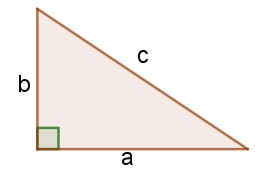### Using the Hypotenuse Formula for Circles

To apply the formula shown in the previous section to find the equation of a circle, one must follow these steps:

1. Preferably, the circle must be drawn on a coordinate system to facilitate finding positions and lengths.
2. Preferably, at first, the center of the circle should coincide with the origin (0, 0) of the coordinate system.
3. A segment r (for radius) must be drawn from the center of the circle and connected to any point over its circumference.
4. A second segment (y) must connect the point of the circumference used in the previous step and connect this point to the x-axis perpendicularly.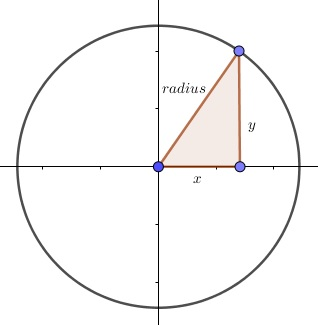Figure 4 shows how a segment from the x-axis completes the figure of the right triangle.

Substituting these values in the formula {eq}a^2 + b^2 = c^2 {/eq}, being {eq}r = c {/eq} (the hypotenuse), the result is:

{eq}x^2 + y^2 = r^2 {/eq}

This is the equation of a circle with a center located at the origin of a coordinate system. Any points (x, y) that satisfy the equation of a circle with radius r, are part of the circumference of this circle.

### Circles Not on the Origin

When the center of a circle is displaced from the origin (0, 0) to a different position (A, B), the difference in the position from each axis must be discounted from the terms x and y of the equation given in the previous section. Thus:

{eq}(x-A)^2 + (y-B)^2 = r^2 {/eq}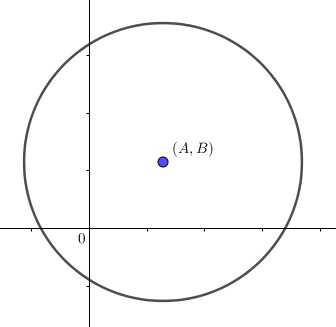### Circle Formula: Standard Form

The previous sections showed how to find the standard equation of a circle:

• {eq}x^2 + y^2 = r^2 {/eq} for a circle with center in the origin.
• {eq}(x-A)^2 + (y-B)^2 = r^2 {/eq} for a circle with a center out of the origin, at a point (A, B).

The equation of a circle, however, can also be expressed in what is called general form.

### Circle Equation: General Form

The circle equation general form is expressed as:

{eq}x^2 + y^2 + Ax + By - C = 0 {/eq}

Where, considering P (x, y) as a point in the circumference of a circle, some terms of the equation are:

• Ax is the product between the location of P and the location of the center in the x-axis.
• By is the product between the location of P and the location of the center in the y-axis.
• C represents the constants (terms without variables).

To unlock this lesson you must be a Study.com Member.

#### How do you find the equation of a circle given two points?

Considering that one of the points is the center and the other is a point on the circumference, one must find the distance between the points (radius). Then, one needs to substitute r for the value of the radius and A and B for the coordinates of the center.

#### How do you find the equation of a circle with the center and radius?

The standard form of the equation will be given by the sum of two terms equals the square of the radius. The first term will be the square of the subtraction (x-A), being A the x-coordinate of the center. The second term will be the square of the subtraction (y-B). Where B is the y-coordinate of the center.

#### How do you find the equation of a circle on a graph?

Choose a point (x, y) on the circumference. Trace a segment connecting the center and the chosen point (radius). Trace a vertical line connecting the point on the circumference and the x-axis. A right triangle will be formed with the hypothenuse being the radius, and the two other sides having the distance x and y. Finally, one simply needs to substitute these variables on the formula of the Pythagorean theorem.

### Register to view this lesson

Are you a student or a teacher?